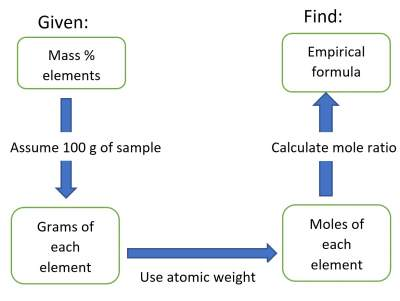# Empirical Formula from Analysis

## Introduction

### Example

• 1 molecule of H2O contains 2 H atoms & 1 O atom.
• 1 mol of H2O contains 2 mol of H atoms and 1 mol of O atom.
• Hence, if the number of moles of the atoms are known, number of moles of their molecule can be determined.
The procedure is shown below:Example: Hg reacts with Cl forming a compound that is 73.9 % Hg and 26.1% Cl by mass.

Question 1: What is the empirical formula of the compound? (Note: Atomic weights of Hg and Cl are 200.6 and 35.5, respectively).

Solution:
• These two elements form ONE compound, whose formula is to be determined.
• The question is about the Empirical Formula; the simplest formula of this compound Procedure
• Assume 100 g sample, this contains: 73.9 g Hg & 26.1 g Cl
• Use the atomic weights of Hg and Cl, to calculate their number of moles: For Hg: # moles = (73.9 / 200.6) = 0.37
• For Cl: # moles = (26.1 / 35.5) = 0.74
• Calculate the molar ratio as follows: Hg : Cl (0.37 : 0.74)
• Divide all numbers by the smallest # of moles Hg : Cl (1 : 2)
Hence, the empirical formula is HgCl2

Question 2: What is the relation between: a "Molecular Formula" and an "Empirical Formula"?